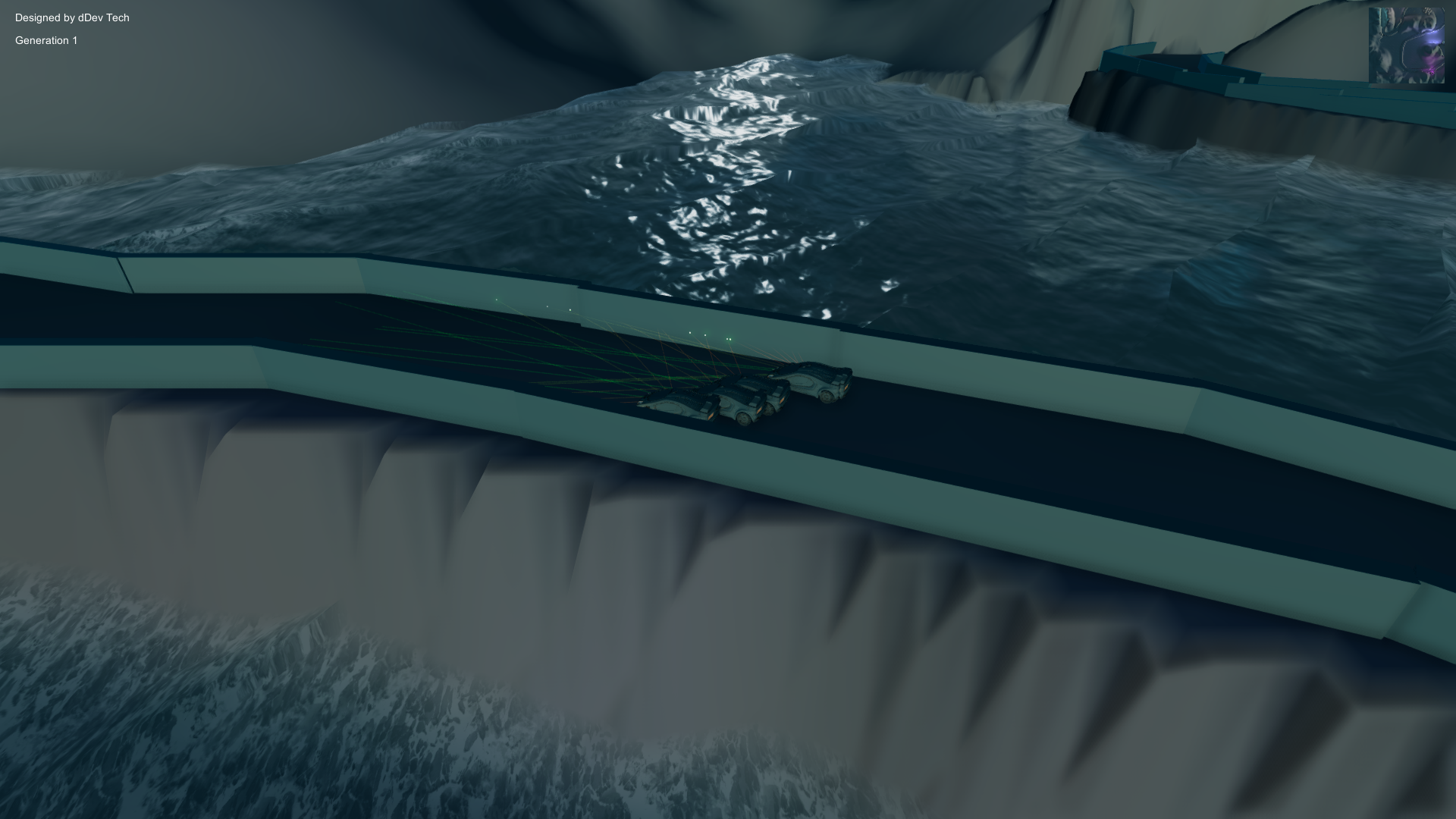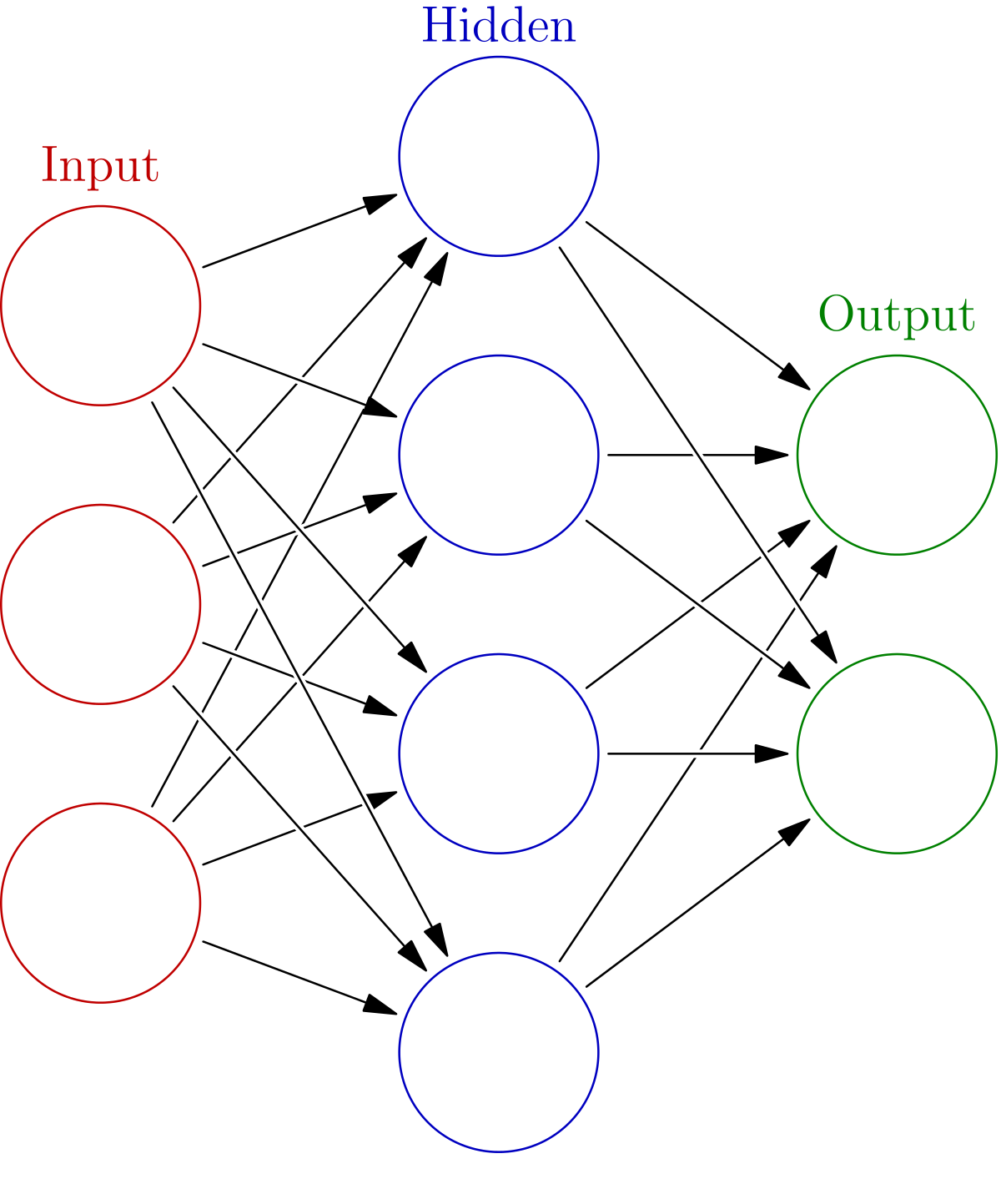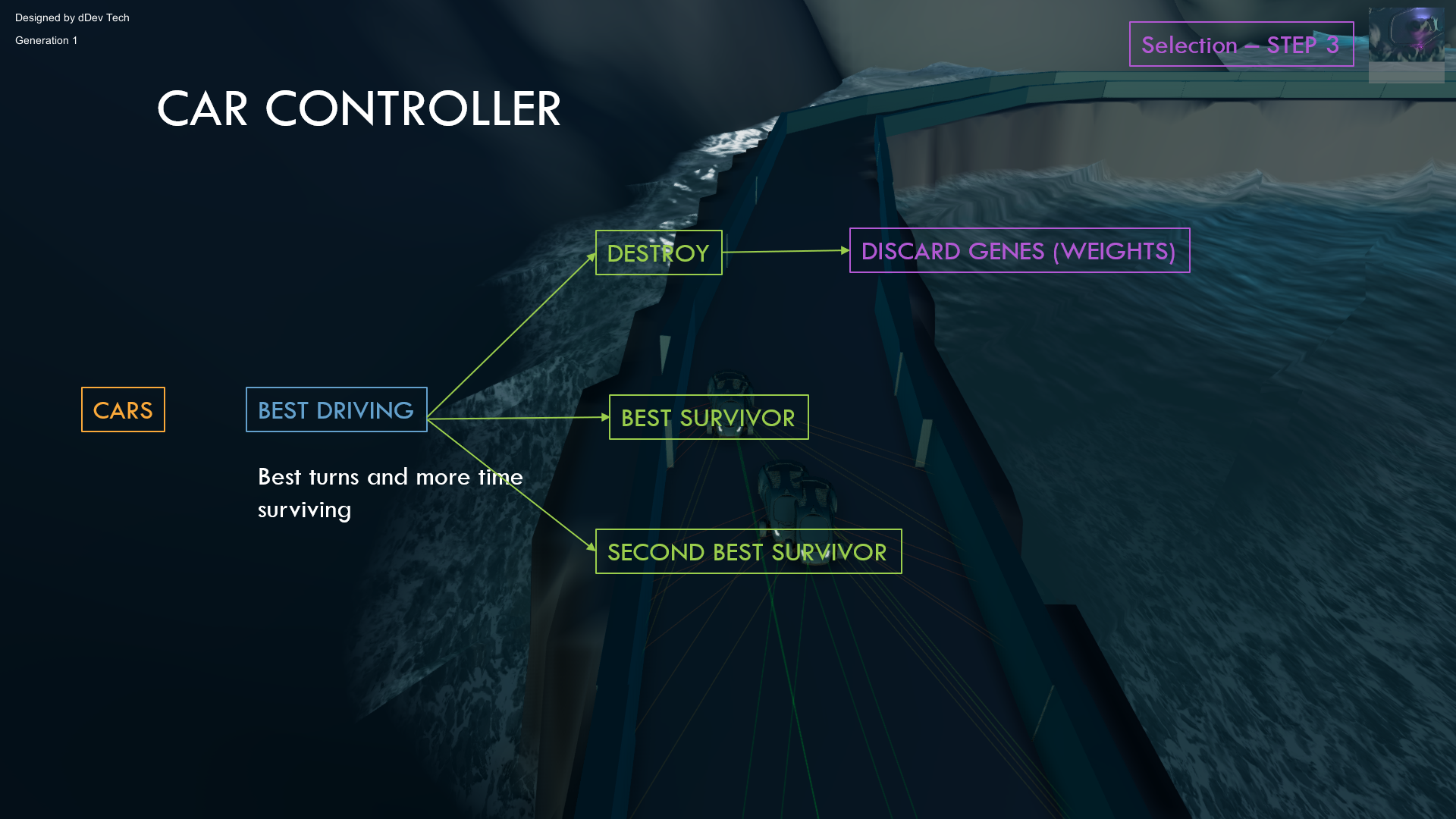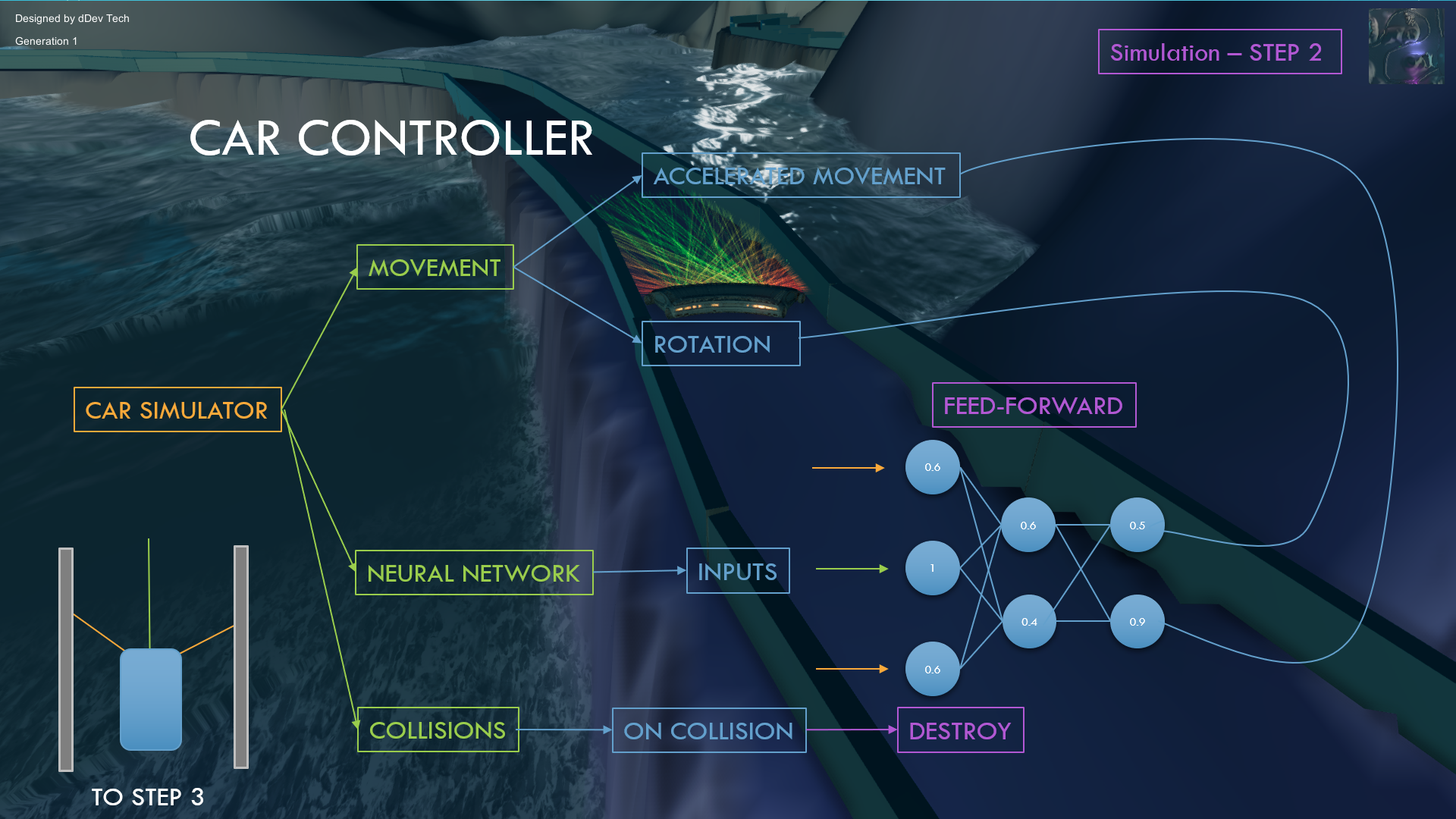SELF DRIVING CAR SIMULATOR
INTRODUCTION

In this tutorials you will learn how to create a self driving simulator where the cars learn to drive using Darwin's law of genetic

The project is created in Unity 3D using C# as programming language. You will learn how to design the map in Unity and the algorithms we implement. Also you will be able to program this algorithms in C#

The project can be divided in different aspects we will must focus on.

• Neural Network: we will be creating a multilayer neural network
• Genetic Algorithm with its respectives crossover and mutation functions
• Visual simulator in 3D with Unity
• Controller: handles all the executions and the algorithms
UNDERSTANDING THE PROJECT

This video explains how all the components work. There are explained the Neural Network, Genetic Algorithm, Camera and Cars.
I explain the neccessary maths and algorithms for the simulator

NEURAL NETWORK

Neural Networks are computing systems that are used to relate different stimulus with different actions or possible solutions. A neural network is organized in layers and each layer will have a certain amount of neurons. This neurons will be connected to others with weights. Every neuron and weights will store a value. The weight values will be static when the network is being updated in our project. However, the neurons values will be changing during the simulator because they will depend on the input layer and the weights of the network.In out project each car will have its own neural network and it will be updated every time. This updated algorithm is called feed-forward. The cars will have as the input layer the distances to the walls that surround the cars. With this inputs and the weights we will get some ouputs that would be the rotation and the acceleration.

We will be using the next formula for updating the network (feed-forward).
Notation: a Activated neuron l Current layer w Weight in layer l that connects the neuron n with m. Bias b added to the multiplication. $$a_m^{l+1} = \sigma(\sum_{n=0}^c{w_{m,n}^{l} a_n^{l}+ b_n^l})$$ The activation function sigma will be the sigmoid function: $$\sigma(x) = \frac{1}{1+e^{-x}}$$
We will apply this to every single neuron starting from the first layer to the last layer or outputs.
Then we will need to put this into practice and create a class in C# called Neural Network that will contain as variables the List of float[][] of weights and the List of neurons organized in layers. It will have two contructors. One for the first generation of cars that will be random weights and the second for assigning the DNA to the weights. It will also have the feed-forward algorithm implemented. You can learn how to program the Neural Network in the next tutorial:

GENETIC ALGORITHM

Instead of using backpropagation for the Neural Network to learn, we will be using reinforcement learning. We will use the Genetic Algorithm applied to every cars. As the Darwin's law says the entity (car) with the best genes will survive and it will propagate the genes to the next generation. They will evolve in a better way and survive more time.

If we want to express this matematically for out cars we should then start by creating for each car is own list of genes. There will be as much genes as the quantity of weights in the neural network of each car. When we create the array we will start by creating it in a random way in the first generation.
We can divide the Genetic Algorithm in different sections:

• Random Initialization of DNAs
• Selection of best cars depending on the fitness function
• Crossover of the genes of the parents
• Mutation the new DNA
INITIALIZATION

The initialization of the DNAs in a random way will be executed only in the first generation when the Neural Networks of the cars are created. We will create the DNA as a List of length the amount of weights in Neural Network. We will create genes in the interval of [-1,1]We will create different random DNAs for each car. Then we will assign this DNA to the weights of the Neural Networks of the cars.

SELECTION

When the simulator finished because all the cars have collided with a wall, we should choose which have the best genes. You can do this in a lot of different ways. Depending on the distance traveled, the average speed or the time driving. I choosed for this project the time of the car driving, so we will ge the genes for the first and second car that survive more time in the track.
All this factors could be joined to obtain a better selection of parents. It can depend also on the diversity we want to achieve.
We could create a function that depends on the factors mentioned before in order to get a specific score for each car called fitnessCROSSOVER

With the parents selected we will swap the genes to create a new DNA with a mix of both parents prefering random fragments from one parent and the other. This random value could be modificated depending if the parents have very different fitness or very similar. As they a similar fitness score they will have more similar probability to be chosen the genes.

You can see here and example of the genes being manipulated:MUTATION

When we create for each new car for the new generation the mixture between both parents we will create different mutations. This is used because the parents are the best from the generation but not the best driving cars. We must create small mutations in the DNA of each new car to get better cars. However we could also get worse car but we will discard for the next generation.

We will define a variable called initial mutation probability that will be how probable is any gen to be mutated. Also this probability will change in an inversily proportional way to the total fitness of all the cars.

$$mutationProbability = \frac{initialProbability}{\sum_{n=0}^{cars}{fitness}}$$

The tutorial of how to program the genetic algorithm and implement the genes in code is the next video:

VISUAL 3D SIMULATOR

Additionaly from the algorithms, we should create all the mesh from the cars, terrain, effects, track and camera.

Each car must be able to move and rotate. Each car will have its own lasers that raycast the distances to the track walls and output a value from 0 to 1.

The camera will behave as a drone that follows one car and will have a smooth movement when changing to other car. It will rotate the cars it is looking to

The terrain, track and effects can be choose by the creator. Nevertheless I have created a video explaining one simple terrain with a track and water:

CONTROLLER

We will finally need to join and interact with all the stuff created before. We will have a class called Controller that will be in charge of controlling every object and algorithm in the simulator.

The lasers will be the inputs of the Neural Network and will be assigned to the input layer of each car with its own lasers. Then we will need to apply the feed-forward algorithm and get the outputs of the Neural Network. These outputs will be the rotation and acceleration of each car that will be used in the physics of the car. This sequence will be updated every time for every car.